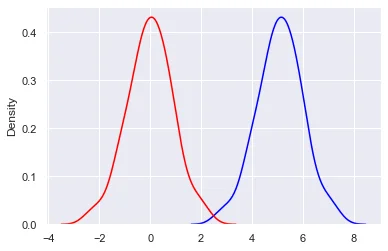03 May 2022

Posted on:

02 May 2022

1

# Addition and Subtraction of the graph (Standardizing a Normal Distribution)

For g(x) = f(x+k) where k >0, Should the graph be shifted to the left, instead to the right? thank you.

e.g. f(x) = x^2, f(2)=f(-2)=4, f(3)=f(-3)=9.
If k = 100 such that g(x) = f(x+100) = (x+100)^2 ,
g(x) = 4 => x = -98 or -102 and g(x) = 9 => x = -97 or -103
g(x) is on the left side of f(x)

Instructor
Posted on:

03 May 2022

2

Hey,

Thank you for the great question! I understand that the lecture might be a bit misleading.

What you are saying is absolutely correct. For a parameter k > 0, a transformation of the type f(x+k) would shift the function to the left while f(x-k) would shift it to the right. The easiest way to understand why this happens is to look at how the y = 0 point moves as the argument of the function changes. Taking your example, f(x) = x^2, the function equals 0 for x = 0. On the other hand, the function f(x-2) equals 0 for x = 2. Therefore, the function moves to the right, as you correctly said.

This situation is a bit different from the one described in the lecture. Let's take the following set of datapoints:
{10, 20, 20, 30, 30, 30, 40, 40, 50}
The distribution of these points will be centered at the mean 30. Now, subtracting the mean from all datapoints yields the following new set of datapoints:
{-20, -10, -10, 0, 0, 0, 10, 10, 20}
which are now centered at 0, that is, to the left of our original distribution.

Here is a short Python script that you might find useful (you will need the packages `numpy`, `matplotlib` and `seaborn` to run it):

``````from numpy import exp, arange
from numpy.random import normal, seed
import numpy as np
import matplotlib.pyplot as plt
import seaborn as sns

sns.set()

seed(1)

data = normal(loc = 5.0, size = 200)
mean = np.mean(data)
data_st = data - mean

fig, ax = plt.subplots()

sns.kdeplot(data, ax = ax, color = "blue")
sns.kdeplot(data_st, ax = ax, color = "red");
``````

In this script, we generate a set of 200 normally distributed points centered around 5.0. This distribution is plotted in blue. We then subtract the mean 5.0 from every datapoint and plot the new distribution in red. It has indeed shifted to the left by 5.0 units because all datapoints are 5.0 units smaller than the original points.